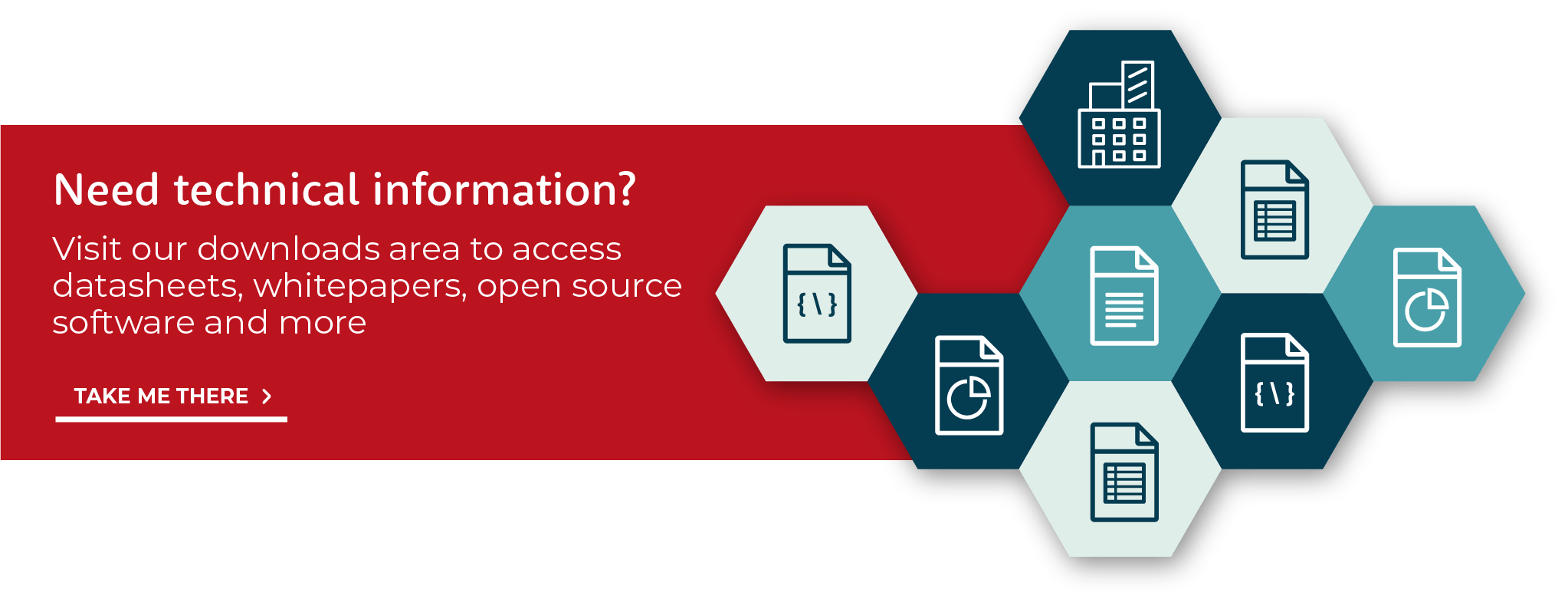AccelerComm CTO Dr Rob Maunder discusses why we need channel coding in wireless communications, and gives an overview of how it works.Hello, I'm Rob Maunder, I'm the CTO of AccelerComm, I'm just gonna talk to you a bit about wireless communications, and the need for error correction.

In wireless comms, we typically have some bits that we're trying to transmit from a transmit antenna over to a receive antenna across a wireless channel. The wireless channel typically imposes impediments like noise, interference, and poor signal, but in spite of those, what we're trying to do is to get back to the original bits over here.

The challenge of all these impediments is that the received signal is typically different to the transmitted signal, so as to address this, we use channel coding. LDPC is an example of this, so in the transmitter, we can use an LDPC encoder, and what this does is it will take a message, which might comprise a number of bits A, and increase the number of bits to a bigger number E. Then the modulator can modulate these bits onto the channel, and the demodulator can produce an output here, which comprises E outputs corresponding to these bits.

These outputs are typically expressed in the form of LLRs, logarithmic likelihood ratios. Now logarithmic likelihood ratios, they don't express the value of the bit, they express the probability of the bit. The reason for this is, because the demodulator can never be sure what noise, interference, poor signal strength, the signal has encountered during the channel. All it can do is it can make a best guess, and then express a probability to go with that guess.

The LDPC decoder has the job of taking these E LLRs corresponding to these E bits here, and converting them back into the A number of bits here, but this job is significantly more complicated than the LDPC encoder's job.

There are a number of challenges here. The first challenge is that these LLRs, they're probabilities, so they're typically expressed as fixed-point numbers, so for every bit that the encoder has to deal with, the decoder has to deal with a probability, a fixed-point number. That makes it more complicated. Also, the encoder knows the value of all the bits that it's dealing with. By contrast, the decoder doesn't know what the message is going to be, that's the whole job of the decoder, is to find out the message, it has to discover what the message is. That makes it more complicated.

The third way that the code is more complicated is that typically, the way that it works, is that it makes an attempt, then another one, then another one, and it iterates several attempts in this way, particularly in the case of LDPC, also turbo codes work this way. So because of these three reasons, LDPC decoders are much more complicated than LDPC encoders.

---

We have produced data briefs that characterise the performance of the decoder across a wide variety of different scenarios.

These can be found within our resources section.